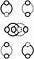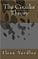The Circular Theory: Core Dynamic in Nature

Explains physics, philosophy, psychology, economics, and politics. Biology and technology. Identity. Complementarity.

Conservation of the Circle: Core Dynamic in Nature

Zero and one is circumference and diameter. Literally. And, figuratively.

Rotation, revolution, and, radiation, involve the conservation of a circle. Any zero and one is, necessarily, circumference and diameter. We can prove this, easily, because zero and one (abstract) is zero and one (concrete) (circumference and diameter).

This is the basis for a physical system (the circular relationship between zero and one, abstract and concrete). It looks like this:Circumference and diameter. Abstract and concrete.

We can say that X and Y are zero and one, by simple substitution, proving pi is the basis (background state) for physical (and abstract) (literal and figurative) reality. Meaning duplicity is the basis for a unit, and, vice versa.

Mathematics and Physics

This gives us the basis for mathematics, and, also, physics. Meaning the variable is constant, and the constant is a variable. Also, articulated ‘perpetual motion is absolute zero’ (and vice versa).

Again, a circular relationship between X and Y (zero and one) (circumference and diameter) produces any (and, every) X and-or Y. This proves a circle is conserved, with every observation.

There is a circular relationship separating, and joining, any, and every, observation, and observer. Making observation possible (and probable). This means the number ‘two’ is constant.

Entanglement (Zero and One) (One and Two)

What we see in the diagram is several entangled circles. Connected, and, therefore, separated, X and Y (zero and one) (circumference and diameter). Any string of characters is maximum-minimum zero-and-or-one.

Any part of the diagram, articulates the entire diagram (and vice versa). To understand the diagram fully, you can reduce, and-or expand it (articulate it as) two circles. Connected into one.

Or, if you like, separated into one. There is a forced ambiguity between the words ‘connected’ and ‘separated’ (reduced and-or expanded) (maximum and-or minimum).

Notation and Sequence

You can also, if you like, decide to see the diagram as zero circles, one circle, an infinite number of circles. Also, three, four, five, or six circles. Any number of circles, produces (expands and reduces to) two circles.

This gives us the basis for sequence. Which gives us the basis for position. And, also, then, time (and, space).

This means a hidden circle is the basis for everything.Zero and one. One and two. Two and three.

Complementarity and Identity

Complementarity is the basis for identity because duplicity is the basis for a unit, because a simple circle is conserved. (X and X, X and X’, X and Y)

This means the forced ambiguity in mathematics and physics explains why, in quantum mechanics, we have several systems within a system (sets of two and-or three). A ‘double identity.’

As proven, and illustrated, above, you cannot have one without two, two without three, three without one. This is because a basic circle (universal circularity) is one-two-three (pi-diameter-circumference) continually entangled. The diagram above is both abstract, and, concrete (one and-or two) (two and-or three) (zero and-or one).

Technology and Biology

A mathematician and a physicist can use either, or both, to define, and, thus, analyze, reality (physical and abstract systems). There is a forced circular relationship between physical and abstract systems (again, proven and demonstrated above).

This explains technology and biology. The underlying basis for zero and one.

It also explains survival and reproduction. Conservation and symmetry. The self-sufficient state.

There is a forced circular relationship between any X and-or Y (all systems, disciplines).Technology and biology. Zero and-or one.

Either-Or, Both-And

This means we need ‘and’ for ‘or,’ and vice versa. It also means we need duplication for negation, and, vice versa. Circle as a noun, and circle as a verb. Duplicity as the basis for any unit (unification, unitization).

For physicists, who are used to ambiguity, this describes, and, also, clears up, the problems we are having integrating gravity and relativity. This is because abstract (mind) and concrete (matter) are connected (and separated) by a circle.

This also means, by abstraction, and deduction, pi is the only observer (basis point for everything). Correct name for what a human labels ‘mind.’ (God) (Nature). (Time and-or space.)

This means a hidden circle is the basis for everything.Abstract and concrete. Mind and matter. Time and space.

Dimensionless Coupling Constant: Pi

So, this diagram removes the need for scale, and-or location (where scale always involves location, and vice versa). Which is what we need to do if we want a complete theory of gravitation (and relativity) (theory of everything).

In simple terms, everything is relative to everything else, and this explains both gravity and relativity. Independent of the size of the circles, above, or, where they are ‘located,’ or, what they are called, the above diagram illustrates circumference and diameter in a way a physicist (any ‘one’) can understand in order to remove location and scale.

This is proven, easily, because only a circle can circle (rotate, revolve, radiate). Integrating all of the aspects of ‘physics,’ including ‘life’ (repetition, reproduction, sequence) (basis for true and-or false).

Constant and Variable

In the world of mathematics, we assume the number line is constant, when, all of us know, this is not completely ‘true.’ ‘True’ and ‘false,’ like any X and Y, articulate, and thus, conserve, a circle. As do the numbers ‘one’ and ‘two’ (zero and one) (all of the numbers on a ‘number’ line).

Thus, the only constant in nature is the conservation of a circle (more accurately labeled number ‘two’). Also, reproduction. Complementarity. And, identity. Where any ‘unit’ articulates, and is dependent on, the number ‘two.’ Giving us the basis for a string (open and-or closed).

This allows us, now, to, rearrange our understandings in physics to incorporate the conservation of a circle as the basis for both electricity and magnetism (radiation and rotation). Which in turn allows us to better understand light (infinite line) and sound (infinite circle). Where all of these are dependent on the zero and the one (circumference and diameter). Where from pi’s point of view the zero is a one (circumference is diameter).

Line and Circle (One and Zero) (Zero and Infinity)

Where line and circle are one and zero, circumference and diameter. Nothing more. Or, less.

Only a circle can create a circle (because a circle can only ‘circle.’) Giving us the basis for a noun, and a verb (language) (movement) (stillness). Abstract and concrete.

It is not possible to understand the concrete without the abstract because concrete and abstract (literal and figurative) are X and Y (zero and one), diameter and circumference, of a, necessarily, conserved circle. Where X and X is, always, X and Y, because zero and one is, always, circumference and diameter (universal ‘unit’).

This is what allows us to abstract nature as an abstract (virtual) identity (virtual persona). Starting (and ending) at ‘square one.’ (Absolute relativity.)Line and circle. Constant and variable. One and zero. Zero and infinity. Female and male. (Universal universal.)Universal ‘unit.’https://www.amazon.com/Circular-Theory-Ilexa-Yardley/dp/0972575626

Written by

The Circular Theory

Conservation of the circle is the core dynamic in Nature.

Welcome to a place where words matter. On Medium, smart voices and original ideas take center stage - with no ads in sight. Watch
Follow all the topics you care about, and we’ll deliver the best stories for you to your homepage and inbox. Explore
Get unlimited access to the best stories on Medium — and support writers while you’re at it. Just \$5/month. Upgrade